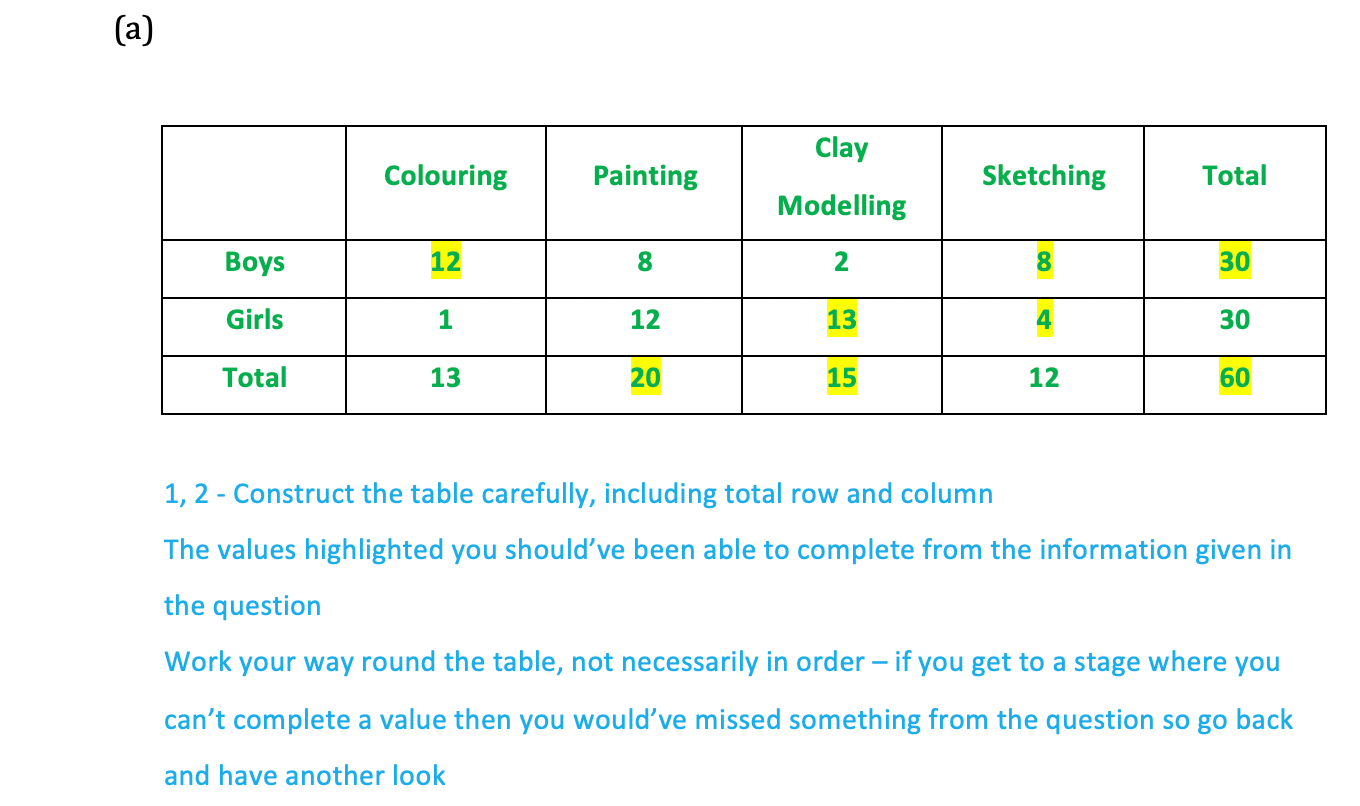# Edexcel IGCSE Maths 复习笔记 6.5.2 Probability - Two Way Tables

Edexcel IGCSE Maths 复习笔记 6.5.2 Probability - Two Way Tables

#### What are two-way tables?

• While Venn diagrams are great at showing overlap they can only show one feature (characteristic) of a situation at one time
• In the notes on Venn diagrams we had an example talking about students studying either Spanish or German, or bothHowever we may also be interested in how many boys and girls were studying Spanish and/or German as well
• This is where we need a two-way table – one of the characteristics will be the columns and the other will be presented in rows
• Once we have our table we can use the numbers within to determine probabilities

#### What do I need to know?

• You’ll need to be able to construct a two-way table from information given in words and then use a table to calculate probabilities
• So you’ll need to be familiar with the basics and notation around probability

1. Total row/column

• It may not be obvious from the wording but a total row and column can be really helpful in two-way table questions
• If they’re not mentioned, or included when given a table, add them in

2. Completing a table

• It may not be possible to add numbers to the table from every sentence, one at a time
• You will usually have to combine one piece of information with another in order to fully complete a table

3. Conditional probability

• Two-way tables in particular give rise to using conditional probability
• This can get complicated but with two-way tables, it is usually straightforward to see which parts of the table the question is referring to

#### Worked Example